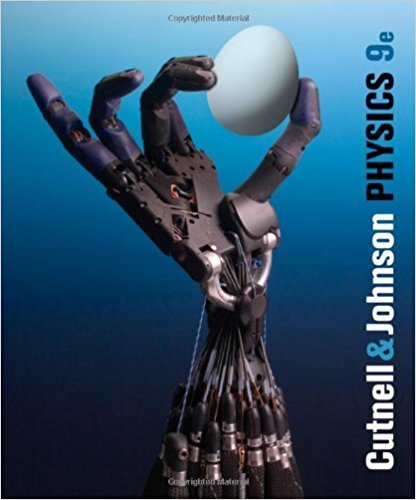×

×

# Solutions for Chapter 4: Forces and Newtons Laws of Motion## Full solutions for Physics, | 9th Edition

ISBN: 9780470879528Solutions for Chapter 4: Forces and Newtons Laws of Motion

Solutions for Chapter 4
4 5 0 290 Reviews
25
1
##### ISBN: 9780470879528

Chapter 4: Forces and Newtons Laws of Motion includes 189 full step-by-step solutions. Since 189 problems in chapter 4: Forces and Newtons Laws of Motion have been answered, more than 201240 students have viewed full step-by-step solutions from this chapter. This textbook survival guide was created for the textbook: Physics,, edition: 9. Physics, was written by and is associated to the ISBN: 9780470879528. This expansive textbook survival guide covers the following chapters and their solutions.

Key Physics Terms and definitions covered in this textbook
• //

parallel

• any symbol

average (indicated by a bar over a symbol—e.g., v¯ is average velocity)

• °C

Celsius degree

• °F

Fahrenheit degree